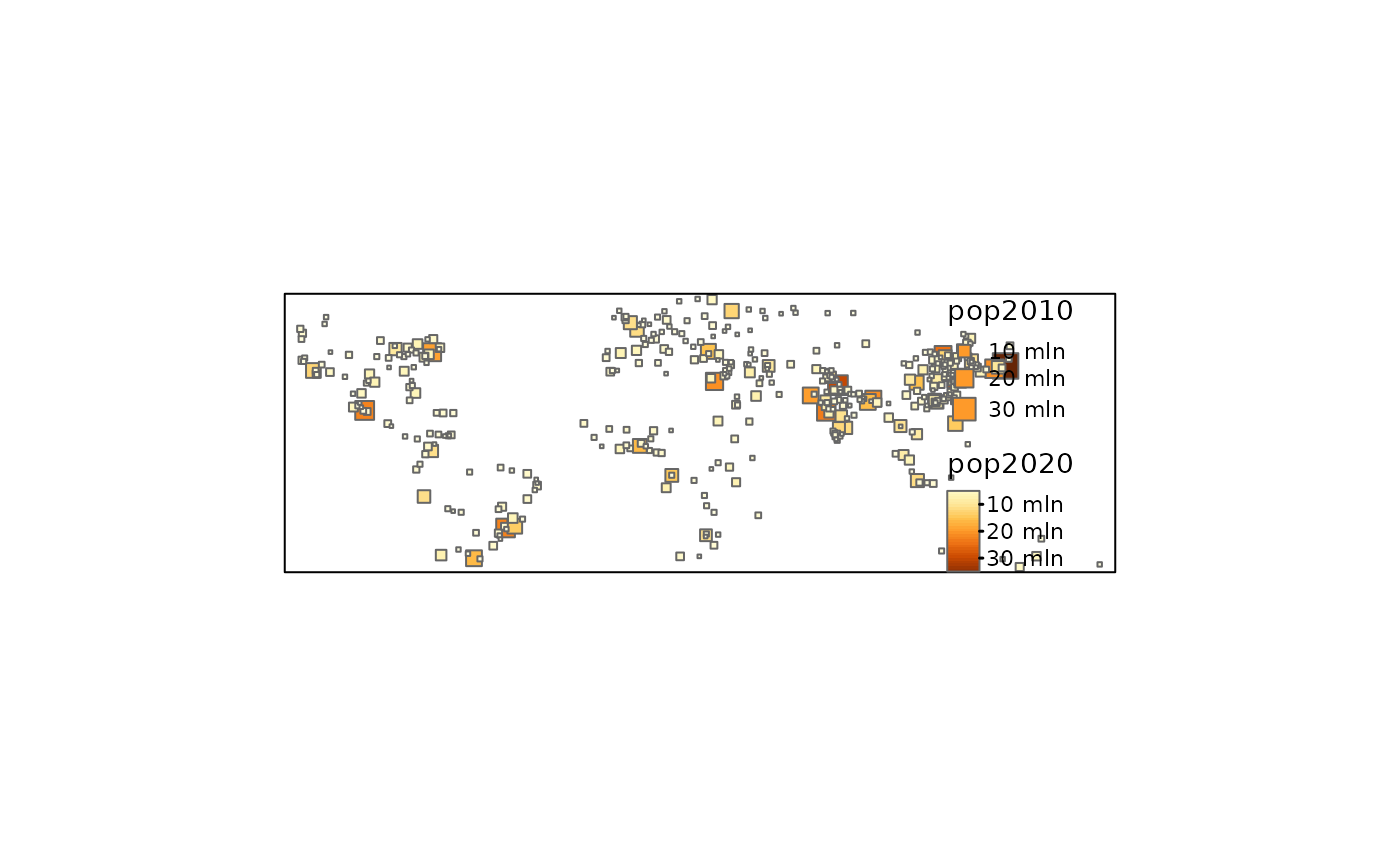Map layer that draws symbols Supported visual variables are: fill (the fill color), col (the border color), size the symbol size, shape the symbol shape, lwd (line width), lty (line type), fill_alpha (fill color alpha transparency) and col_alpha (border color alpha transparency).

## Usage

tm_symbols(
size = tm_const(),
size.scale = tm_scale(),
size.legend = tm_legend(),
size.free = NA,
fill = tm_const(),
fill.scale = tm_scale(),
fill.legend = tm_legend(),
fill.free = NA,
col = tm_const(),
col.scale = tm_scale(),
col.legend = tm_legend(),
col.free = NA,
shape = tm_const(),
shape.scale = tm_scale(),
shape.legend = tm_legend(),
shape.free = NA,
lwd = tm_const(),
lwd.scale = tm_scale(),
lwd.legend = tm_legend(),
lwd.free = NA,
lty = tm_const(),
lty.scale = tm_scale(),
lty.legend = tm_legend(),
lty.free = NA,
fill_alpha = tm_const(),
fill_alpha.scale = tm_scale(),
fill_alpha.legend = tm_legend(),
fill_alpha.free = NA,
col_alpha = tm_const(),
col_alpha.scale = tm_scale(),
col_alpha.legend = tm_legend(),
col_alpha.free = NA,
plot.order = tm_plot_order("size"),
trans.args = list(points.only = "ifany"),
mapping.args = list(icon.scale = 3, just = NA, grob.dim = c(width = 48, height = 48,
render.width = 256, render.height = 256)),
zindex = NA,
group = NA,
group.control = "check",
popup.vars = NA,
popup.format = list(),
hover = "",
id = "",
...
)

tm_dots(
fill = tm_const(),
fill.scale = tm_scale(),
fill.legend = tm_legend(),
fill.free = NA,
size = tm_const(),
size.scale = tm_scale(),
size.legend = tm_legend(),
size.free = NA,
lwd = tm_const(),
lwd.scale = tm_scale(),
lwd.legend = tm_legend(),
lwd.free = NA,
lty = tm_const(),
lty.scale = tm_scale(),
lty.legend = tm_legend(),
lty.free = NA,
fill_alpha = tm_const(),
fill_alpha.scale = tm_scale(),
fill_alpha.legend = tm_legend(),
fill_alpha.free = NA,
plot.order = tm_plot_order("size"),
trans.args = list(points.only = "ifany"),
mapping.args = list(icon.scale = 3, just = NA, grob.dim = c(width = 48, height = 48,
render.width = 256, render.height = 256)),
zindex = NA,
group = NA,
group.control = "check",
...
)

tm_bubbles(
size = tm_const(),
size.scale = tm_scale(),
size.legend = tm_legend(),
size.free = NA,
fill = tm_const(),
fill.scale = tm_scale(),
fill.legend = tm_legend(),
fill.free = NA,
col = tm_const(),
col.scale = tm_scale(),
col.legend = tm_legend(),
col.free = NA,
lwd = tm_const(),
lwd.scale = tm_scale(),
lwd.legend = tm_legend(),
lwd.free = NA,
lty = tm_const(),
lty.scale = tm_scale(),
lty.legend = tm_legend(),
lty.free = NA,
fill_alpha = tm_const(),
fill_alpha.scale = tm_scale(),
fill_alpha.legend = tm_legend(),
fill_alpha.free = NA,
col_alpha = tm_const(),
col_alpha.scale = tm_scale(),
col_alpha.legend = tm_legend(),
col_alpha.free = NA,
plot.order = tm_plot_order("size"),
trans.args = list(points.only = "ifany"),
mapping.args = list(icon.scale = 3, just = NA, grob.dim = c(width = 48, height = 48,
render.width = 256, render.height = 256)),
zindex = NA,
group = NA,
group.control = "check",
...
)

## Arguments

size, size.scale, size.legend, size.free

Visual variable that determines the size. See details.

fill, fill.scale, fill.legend, fill.free

Visual variable that determines the fill color. See details.

col, col.scale, col.legend, col.free

Visual variable that determines the col color. See details.

shape, shape.scale, shape.legend, shape.free

Visual variable that determines the shape. See details.

lwd, lwd.scale, lwd.legend, lwd.free

Visual variable that determines the line width. See details.

lty, lty.scale, lty.legend, lty.free

Visual variable that determines the line type. See details.

fill_alpha, fill_alpha.scale, fill_alpha.legend, fill_alpha.free

Visual variable that determines the fill color alpha transparency See details.

col_alpha, col_alpha.scale, col_alpha.legend, col_alpha.free

Visual variable that determines the border color alpha transparency. See details.

plot.order

Specification in which order the spatial features are drawn. See tm_plot_order() for details.

trans.args, mapping.args

lists that are passed on to internal transformation and mapping functions respectively

zindex

Map layers are drawn on top of each other. The zindex numbers (one for each map layer) determines the stacking order. By default the map layers are drawn in the order they are called.

group

Name of the group to which this layer belongs. This is only relevant in view mode, where layer groups can be switched (see group.control)

group.control

In view mode, the group control determines how layer groups can be switched on and off. Options: "radio" for radio buttons (meaning only one group can be shown), "check" for check boxes (so multiple groups can be shown), and "none" for no control (the group cannot be (de)selected).

popup.vars

names of data variables that are shown in the popups in "view" mode. Set popup.vars to TRUE to show all variables in the shape object. Set popup.vars to FALSE to disable popups. Set popup.vars to a character vector of variable names to those those variables in the popups. The default (NA) depends on whether visual variables (e.g.col) are used. If so, only those are shown. If not all variables in the shape object are shown.

popup.format

list of formatting options for the popup values. See the argument legend.format for options. Only applicable for numeric data variables. If one list of formatting options is provided, it is applied to all numeric variables of popup.vars. Also, a (named) list of lists can be provided. In that case, each list of formatting options is applied to the named variable.

hover

name of the data variable that specifies the hover labels

id

name of the data variable that specifies the indices of the spatial features. Only used for "view" mode.

...

to catch deprecated arguments from version < 4.0

## Details

The visual variable arguments (e.g. col) can be specified with either a data variable name (of the object specified in tm_shape()), or with a visual value (for col, a color is expected). Multiple values can be specified: in that case facets are created. These facets can be combined with other faceting data variables, specified with tm_facets().

The .scale arguments determine the used scale to map the data values to visual variable values. These can be specified with one of the available tm_scale_ functions. The default scale that is used is specified by the tmap option scales.var.

The .legend arguments determine the used legend, specified with tm_legend(). The default legend and its settings are determined by the tmap options legend..

The .free arguments determine whether scales are applied freely across facets, or shared. A logical value is required. They can also be specified with a vector of three logical values; these determine whether scales are applied freely per facet dimension. This is only useful when facets are applied (see tm_facets()). There are maximally three facet dimensions: rows, columns, and pages. This only applies for a facet grid (tm_facets_grid()). For instance, col.free = c(TRUE, FALSE, FALSE) means that for the visual variable col, each row of facets will have its own scale, and therefore its own legend. For facet wraps and stacks (tm_facets_wrap() and tm_facets_stack()), there is only one facet dimension, so the .free argument requires only one logical value.

## Examples

# load Africa country data
data(World, metro)
Africa = World[World$continent == "Africa", ] metro_A = sf::st_intersection(metro, Africa) #> Warning: attribute variables are assumed to be spatially constant throughout all geometries tm_shape(metro_A) + tm_symbols()tm_shape(Africa) + tm_polygons() + tm_shape(metro_A) + tm_symbols(fill = "pop1950", size = "pop2030", size.scale = tm_scale(values.scale = 2))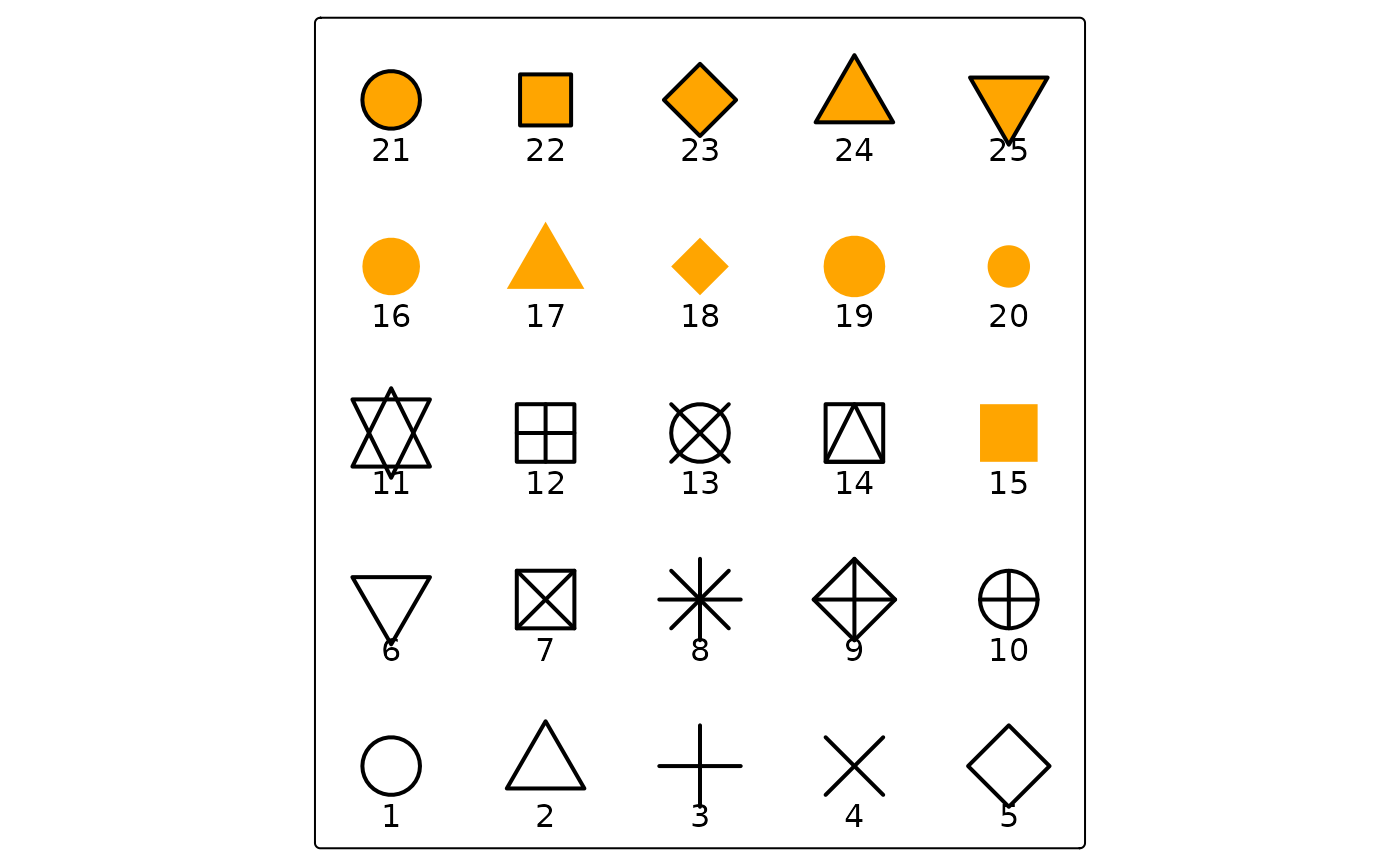##### tmap v3 data(World, metro) metro$growth <- (metro$pop2020 - metro$pop2010) / (metropop2010 * 10) * 100 tm_shape(World) + tm_fill("grey70") + tm_shape(metro) + tm_bubbles("pop2010", col = "growth", border.col = "black", border.alpha = .5, style="fixed", breaks=c(-Inf, seq(0, 6, by=2), Inf), palette="-RdYlBu", contrast=1, title.size="Metro population", title.col="Growth rate (%)") + tm_format("World") #> Deprecated tmap v3 code detected. Code translated to v4 #> Variable(s) "fill" contains positive and negative values, so midpoint is set to 0. Set midpoint = NA to show the full spectrum of the color palette.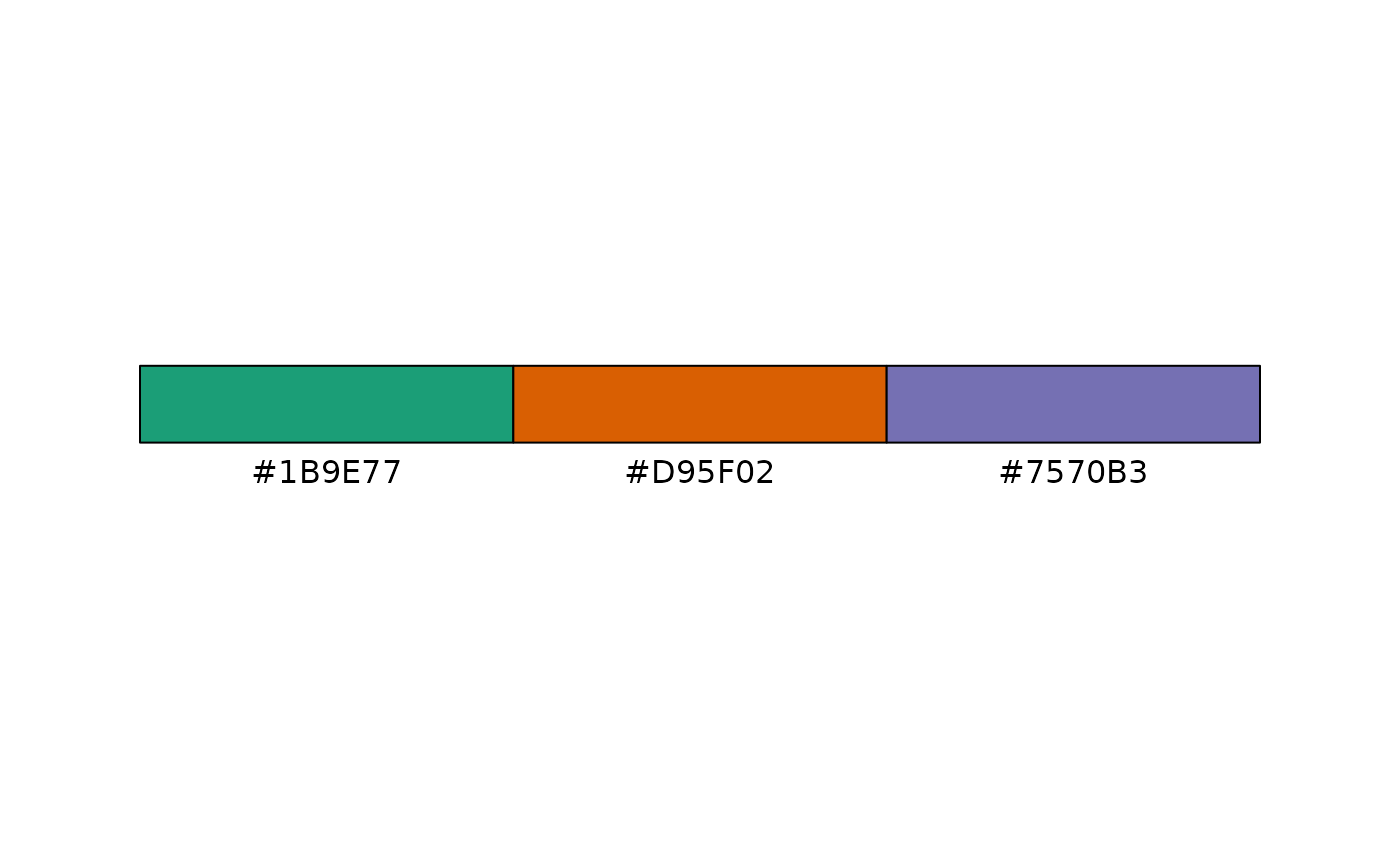tm_shape(metro) + tm_symbols(size = "pop2010", col="pop2010", shape="pop2010", legend.format = list(text.align="right", text.to.columns = TRUE)) + tm_legend(outside = TRUE, outside.position = "bottom", stack = "horizontal") #> Deprecated tmap v3 code detected. Code translated to v4 #> Warning: v3 code detected: as of tmap v4, tm_legend should be specified per visual variable (e.g. with the argument fill.legend of tm_polygonsif (require(ggplot2) && require(dplyr) && require(tidyr) && require(tmaptools) && require(sf)) { data(NLD_prov) origin_data <- NLD_prov %>% st_set_geometry(NULL) %>% dplyr::mutate(FID = factor(dplyr::row_number())) %>% dplyr::select(FID, origin_native, origin_west, origin_non_west) %>% tidyr::pivot_longer( cols = c(origin_native, origin_west, origin_non_west), names_to = "origin", values_to = "perc", names_transform = as.factor ) %>% dplyr::arrange(origin, FID) %>% as.data.frame() origin_cols <- get_brewer_pal("Dark2", 3) grobs <- lapply(split(origin_data, origin_dataFID), function(x) {
ggplotGrob(ggplot(x, aes(x="", y=-perc, fill=origin)) +
geom_bar(width=1, stat="identity") +
scale_y_continuous(expand=c(0,0)) +
scale_fill_manual(values=origin_cols) +
theme_ps(plot.axes = FALSE))
})

names(grobs) <- NLD_prov$name tm_shape(NLD_prov) + tm_polygons(group = "Provinces") + tm_symbols(size = "population", shape="name", shapes = grobs, sizes.legend = c(.5, 1,3)*1e6, scale = 1, legend.shape.show = FALSE, legend.size.is.portrait = TRUE, shapes.legend = 22, title.size = "Population", group = "Charts", id = "name", popup.vars = c("population", "origin_native", "origin_west", "origin_non_west")) + tm_add_legend(type = "fill", group = "Charts", col = origin_cols, labels = c("Native", "Western", "Non-western"), title = "Origin") + tm_format("NLD") grobs2 = grobs grobs2[] = 21 NLD_prov$population[1:5] = 500000
tm_shape(NLD_prov) +
tm_polygons(group = "Provinces") +
tm_symbols(shape="name",
fill = "red",
col = "blue",
size = "population",
size.scale = tm_scale_continuous(values.scale = 4),
shape.scale = tm_scale_categorical(values = grobs2),
shape.legend = tm_legend_hide())

}
#>
#> Attaching package: ‘dplyr’
#> The following objects are masked from ‘package:stats’:
#>
#>     filter, lag
#> The following objects are masked from ‘package:base’:
#>
#>     intersect, setdiff, setequal, union
#> Linking to GEOS 3.10.2, GDAL 3.4.1, PROJ 8.2.1; sf_use_s2() is TRUE
#> Deprecated tmap v3 code detected. Code translated to v4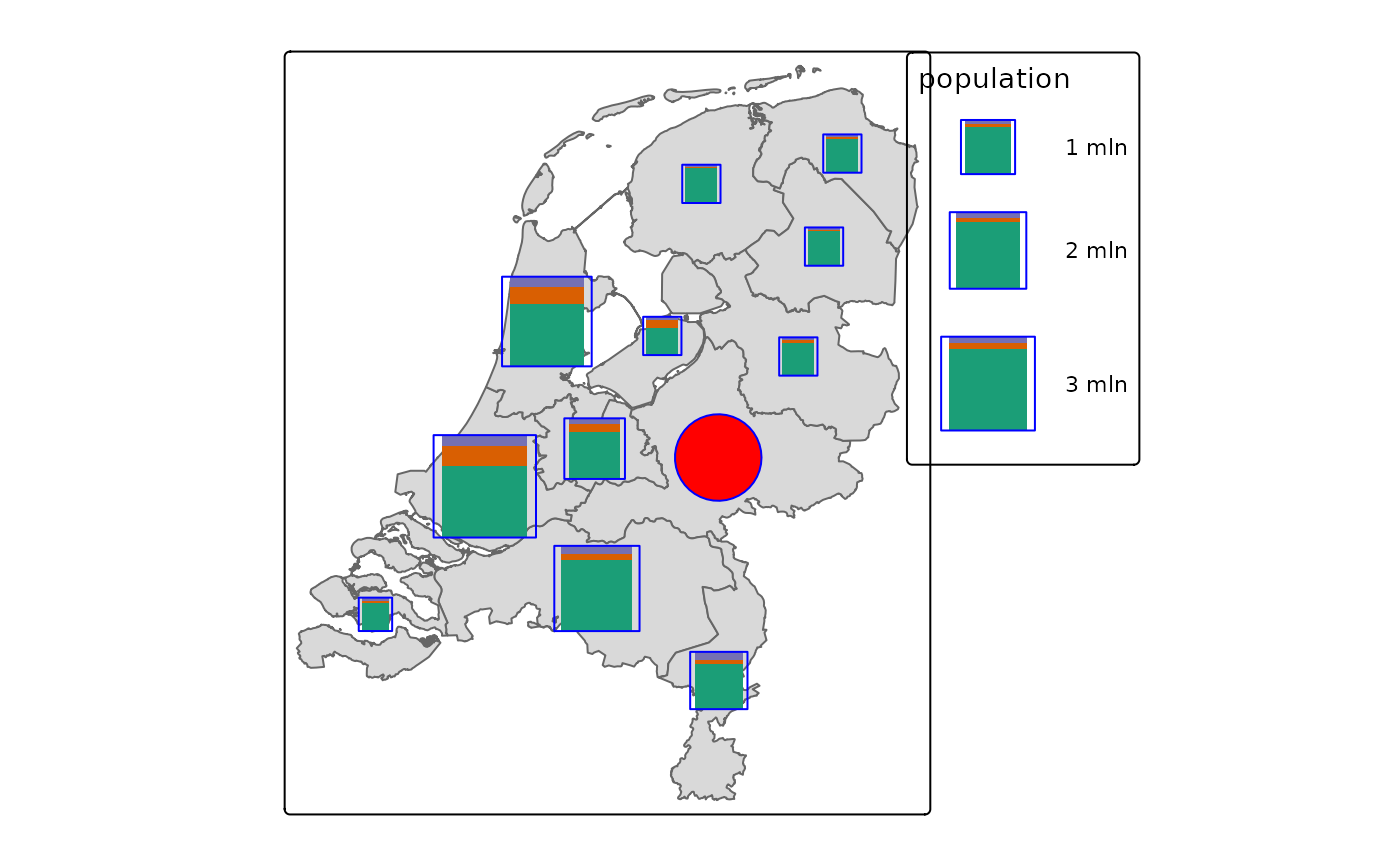# TIP: check out these examples in view mode, enabled with tmap_mode("view")

if (FALSE) {
if (require(rnaturalearth)) {

airplane <- tmap_icons(system.file("img/airplane.png", package = "tmap"))

current.mode <- tmap_mode("view")
tm_shape(airports) +
tm_symbols(shape=airplane, size="natlscale",
legend.size.show = FALSE, scale=1,
border.col = NA, id="name", popup.vars = TRUE)
#+  tm_view(set.view = c(lon = 15, lat = 48, zoom = 4))
tmap_mode(current.mode)
}
}

#####################################################################################

if (FALSE) {
# plot all available symbol shapes:
if (require(ggplot2)) {
ggplot(data.frame(p=c(0:25,32:127))) +
geom_point(aes(x=p%%16, y=-(p%/%16), shape=p), size=5, fill="red") +
geom_text(mapping=aes(x=p%%16, y=-(p%/%16+0.25), label=p), size=3) +
scale_shape_identity() +
theme(axis.title=element_blank(),
axis.text=element_blank(),
axis.ticks=element_blank(),
panel.background=element_blank())
}
}

tm_shape(metro) +
tm_symbols("pop2010", size.scale = tm_scale_continuous(n = 8, values.scale = 3), shape = 22)
#> Warning: Some legend items or map compoments do not fit well (e.g. due to the specified font size).data("NLD_prov")
NLD_prov\$x = seq(10, by = 5, length.out = 12)

tm_shape(NLD_prov) +
tm_symbols(size = "x", size.scale = tm_scale_continuous(values.scale = 4)) +
tm_text(text = "x")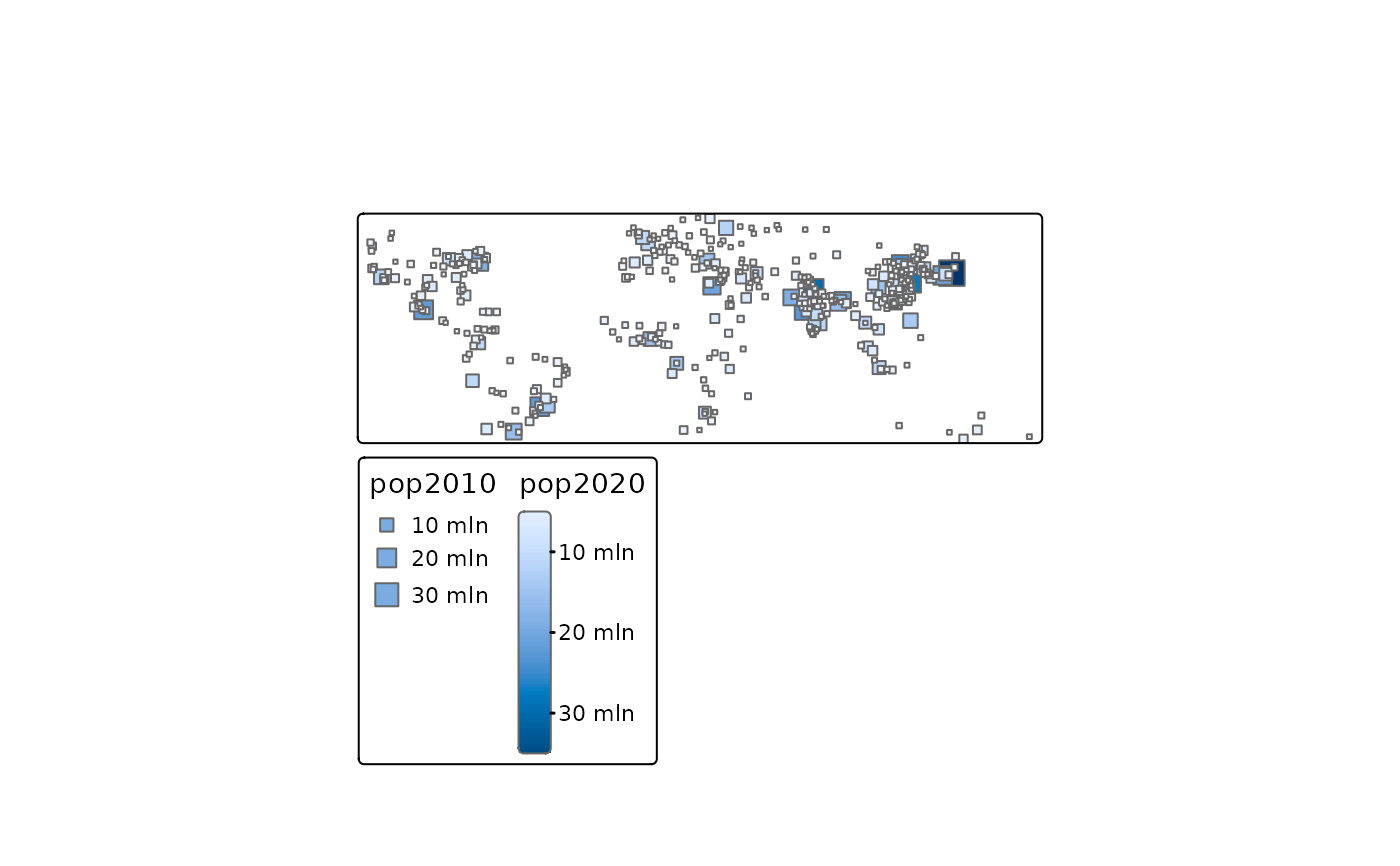tm_shape(metro) +
tm_symbols("pop2010", fill = "pop2020",
fill.scale = tm_scale_continuous(),
size.scale = tm_scale_continuous(), shape = 22)